# CHAR function in ExcelThe CHAR function in Excel returns a specific character based on a code number from the operating system’s character set.

Each operating system has it’s own character set:

• Windows has the Windows -1251 character set, which is more commonly known as ANSI
• Mac uses the Mac OS Roman character set.
• Excel online has a limited version of the Windows character set.

The character set contains the primary printed and non-printed characters available on a computer.  Those characters can be letters, numbers, punctuation, symbols, and even characters that are not displayed (such as line breaks).

Every character in the set has a code.  The CHAR function in Excel returns the character associated with each code.

It is probably most useful when:

• inserting line breaks into a formula
• inserting double quotes into a formula
• combined with SUBSTITUTE to remove non-printed characters

We’ll look at each of these as examples later in this post.

I recommend you download the files which support this post, as you’ll be able to work along with examples.  This is the best way to learn.  You’ll be able to see the solutions in action, plus the file will be useful for future reference.  The support files are available for FREE to newsletter subscribers.

• My favorite tips and tricks direct to your inbox
• Exclusive content (which is only available to subscribers)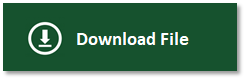The filename for this post is 0045 CHAR function in Excel.xlsx

Contents

## The arguments of the CHAR function

The CHAR function is easy to use.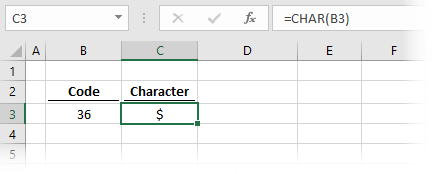The example above uses the character code 36 from cell B3 and converts it into a character.

`=CHAR(B3)`

The CHAR function in Excel has just one argument

`=CHAR(number)`
• number: an integer value from 1 to 255 representing the character to be returned for the operating system’s character set.

Working with different character sets

With each operating system having its own character set, it makes the translation between Excel versions a little tricky.

Windows vs. Mac:

If the spreadsheet is likely to be used on another operating system, we can use the INFO function to determine which operating system is running.

As an example, the line break character is different on Windows and Mac:

• Windows – CHAR(10) is a line break
• Mac – CHAR(13) is a line break

The formula below tests if the operating system is a Mac and assigns CHAR(13) if it is, or CHAR(10) if it is not.

`=IF(INFO("SYSTEM")="mac",CHAR(13),CHAR(10))`

Excel online:

Excel online has a limited character set.  We can only use the codes 9, 10, 13, and 32 onwards.

Don’t worry; you’re not missing out. The missing items are generally non-printed characters that Excel online does not require.

## Examples of using the CHAR function

In this section, we’ll look at some practical examples of using the CHAR function.

### Inserting special characters

The screenshot below shows some of the special characters which are available when using the CHAR function.As can be seen, Code 128 is equivalent to the € symbol; code 153 is equivalent to the Trade Mark ™ symbol, etc., etc.  These are just a small sample of the available characters.

### Create line break in formula

In this example, we have what is probably the most common usage of the CHAR function.

CHAR(10) (or CHAR(13) on a Mac) is equivalent to inserting a new line into a formula.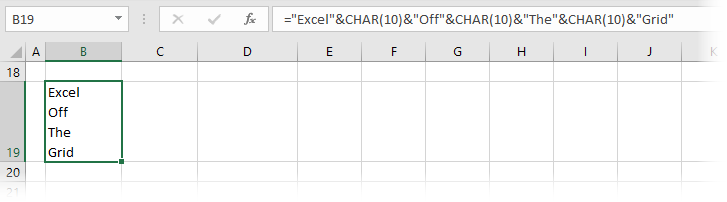In cell B19 in the screenshot above, we have the following formula:

`="Excel"&CHAR(10)&"Off"&CHAR(10)&"The"&CHAR(10)&"Grid"`

The CHAR(10) part of the formula is inserting a line break between each word.  As a result, the value in cell B19 is separated over 4 lines.

The cell needs to be formatted with Wrap Text, else the text will be displayed on a single line without any line breaks.

Instead of using CHAR(10), a line break can also be entered directly into the formula bar by pressing ALT + Enter.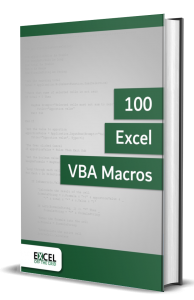• Contains 100 Excel VBA macros
• Learn VBA by following along with the example codes
• Apply to your macros, automate Excel, save time.### Insert double quotes into a text string

Double quotes are used in Excel at the start and end of a text string.  But what if we need to create a string that contains a double quote?  That can be tricky.

The easiest option is to use CHAR(34).  Look at the screenshot below; we have created a sentence that includes two double-quote characters.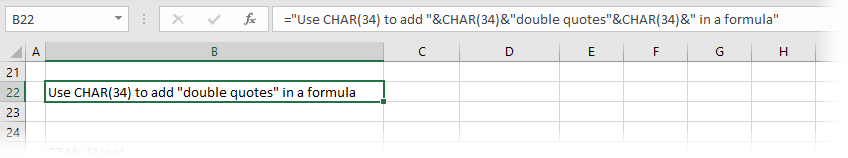The formula in cell B22 is:

`="Use CHAR(34) to add "&CHAR(34)&"double quotes"&CHAR(34)&" in a formula"`

In this formula, we are using & to join text strings together.  CHAR(34) is used to create the double quote.

An alternative approach is to use two double quotes next to each other inside a text string.  The same formula written using this method would be:

`="Use CHAR(34) to add"&" """&"double quotes"" "&"in a formula"`

Look at all those double-quotes, that’s a lot!  Basically, it’s so confusing and difficult to read that you’re better off using CHAR(34).

### Removing non-printed characters

If we have non-printed characters that we want to remove, we can use CHAR combined with the SUBSTITUTE function.

Look at the screenshot below.There is an address in cell B25.  The address includes line breaks.  We can convert it into a comma-separated text string, as seen in cell C25.

The formula in cell C25 is:

`=SUBSTITUTE(B25,CHAR(10),", ")`

This formula replaces every instance of a CHAR(10) and replaces it with a comma and space.

### Using CHAR with VBA

Most functions in Excel can be used within VBA by using code similar to the following:

`Range("A1") = Application.WorksheetFunction.CHAR(36)`

However, the CHAR worksheet function cannot be used within VBA.  However, we can use the VBA only CHR function.

`Range("A1") = Chr(36)`

The Excel CHAR function and the VBA Chr function both operate in the same way.

## Finding the code for a character

The CHAR function is for converting a code into text character, but what if we want the inverse?  In that circumstance, we can use the CODE function.

The formula below will give the result of 36, as that is the character code number for the \$ symbol.

`=CODE("\$")`

Other information

1. If we use CHAR to return a non-printed character, Excel will display a rectanglar placeholder.
2. Most non-printed characters can be removed using the CLEAN function.
3. #VALUE errors occur when the number provided is not an integer between 1 and 255.
4. The UNICHAR function provides access to an extended character set using codes above 255.

## Conclusion

In this post, we’ve seen how to use the CHAR function in Excel.  While at the outset, it may not seem like a useful function, through the examples, we’ve seen that it can help us in many tricky scenarios.

Don’t forget: